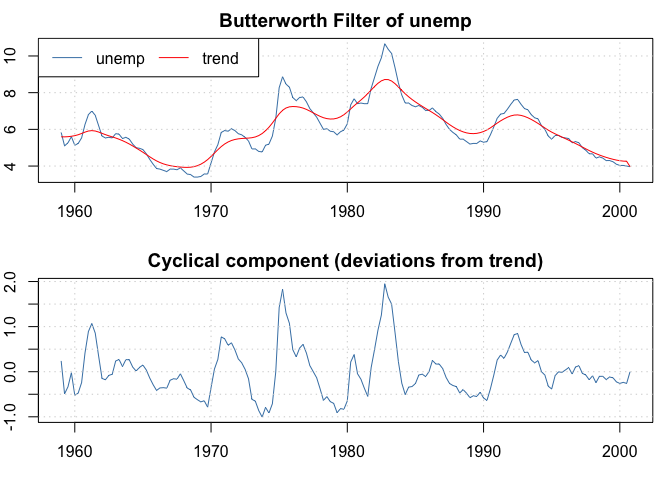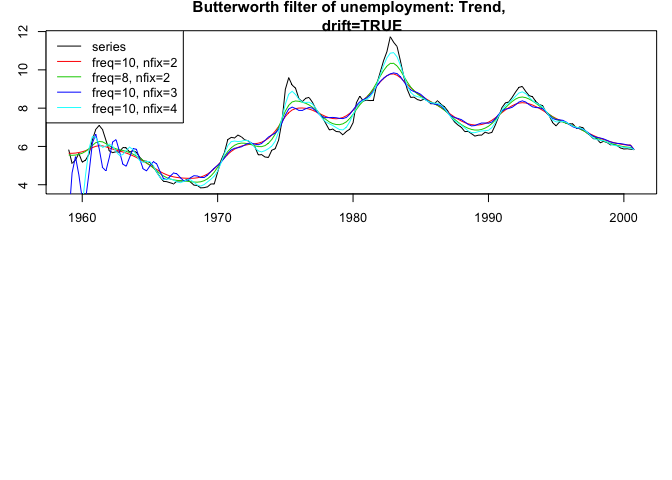# mFilter

The mFilter package implements several time series filters useful for smoothing and extracting trend and cyclical components of a time series. The routines are commonly used in economics and finance, however they should also be interest to other areas. Currently, Christiano-Fitzgerald, Baxter-King, Hodrick-Prescott, Butterworth, and trigonometric regression filters are included in the package.

## Installation

You can install the released version of mFilter from CRAN with:

``install.packages("mFilter")``

The development version can be installed with:

``devtools::install_github("mbalcilar/mFilter")``

## Example

This is a basic example which shows you how to do Butterworth filtering:

``````library(mFilter)
data(unemp)
unemp.bw <- bwfilter(unemp)
plot(unemp.bw)````````````unemp.bw1 <- bwfilter(unemp, drift=TRUE)
unemp.bw2 <- bwfilter(unemp, freq=8,drift=TRUE)
unemp.bw3 <- bwfilter(unemp, freq=10, nfix=3, drift=TRUE)
unemp.bw4 <- bwfilter(unemp, freq=10, nfix=4, drift=TRUE)``````
``````par(mfrow=c(2,1),mar=c(3,3,2,1),cex=.8)
plot(unemp.bw1\$x,
main="Butterworth filter of unemployment: Trend,
drift=TRUE",col=1, ylab="")
lines(unemp.bw1\$trend,col=2)
lines(unemp.bw2\$trend,col=3)
lines(unemp.bw3\$trend,col=4)
lines(unemp.bw4\$trend,col=5)
legend("topleft",legend=c("series", "freq=10, nfix=2",
"freq=8, nfix=2", "freq=10, nfix=3", "freq=10, nfix=4"),
col=1:5, lty=rep(1,5), ncol=1)````````````plot(unemp.bw1\$cycle,
main="Butterworth filter of unemployment: Cycle,drift=TRUE",
col=2, ylab="", ylim=range(unemp.bw3\$cycle,na.rm=TRUE))
lines(unemp.bw2\$cycle,col=3)
lines(unemp.bw3\$cycle,col=4)
lines(unemp.bw4\$cycle,col=5)``````